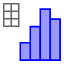# IM3.7B.2 Exit Ticket

Author:
Lind, Jon
A class of 28 eleventh graders wanted to estimate the proportion of all juniors and seniors at their high school with part-time jobs after school. Each eleventh grader took a random sample of juniors and seniors and then calculated the proportion with part-time jobs. Following are the sample proportions. 0.7,0.8,0.57,0.63,0.7,0.47,0.67,0.67,0.8,0.77,0.4,0.73,0.63,0.67,0.6,0.77,0.77,0.77,0.53, 0.57,0.73,0.7,0.67,0.7,0.77,0.57,0.77,0.67 Construct a dot plot of the sample proportions below by 1. entering the values in column A 2. Selecting all of the values and choosing One Variable Analysis3. Change "Histogram" to "Dot plot"

﻿A. Describe the shape of the distribution B. Find the mean and standard deviation of the sample proportions using the "show statistics" button (ask your teacher). C. Do you think that the proportion of all juniors and seniors at the school with part-time jobs could be 0.7? Do you think it could be 0.5? Justify your answers based on your dot plot.D. ﻿a. Suppose the eleventh graders had taken random samples of size 60. How would the distribution of sample proportions based on samples of size 60 differ from the distribution for samples of size 30?